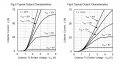# Theoretical calculation of Losses of an IGBT using datasheet information

#### mohtishamali

Joined Jul 9, 2021
55
Hello Everyone,
I want to compute losses of an IGBT using datasheet information given by manufacturer. The problem i have is that I want to calculate losses at 90Cdeg junction temperature while the information provided on datasheet is only for 25Cdeg and 175Cdeg of junction temperature. Could anyone here please guide me how can i calculate the approx. losses for 90Cdeg?
Thanks

#### Papabravo

Joined Feb 24, 2006
19,852
You have to make a mathematical model of the dependence. I assume you know how to do interpolation if the dependence is linear. If it is not linear then the next best alternative is to fit a polynomial; some spice simulators have device models that allow for the modeling of temperature effects. If you have such a model this will be easier than any other approach. In these models there are obvious polynomial approximations that are used.

ETA: The models I have seen use linear and quadratic terms to model temperature effects.

Last edited:

#### mohtishamali

Joined Jul 9, 2021
55
You have to make a mathematical model of the dependence. I assume you know how to do interpolation if the dependence is linear. If it is not linear then the next best alternative is to fit a polynomial; some spice simulators have device models that allow for the modeling of temperature effects. If you have such a model this will be easier than any other approach. In these models there are obvious polynomial approximations that are used.

ETA: The models I have seen use linear and quadratic terms to model temperature effects.
I want to have a curve at VGE=15V and Tj=90Cdeg. It seems linear to me#### Papabravo

Joined Feb 24, 2006
19,852
Well, I guess you could sketch an approximation between the two curves or buy a few parts and do the testing yourself.